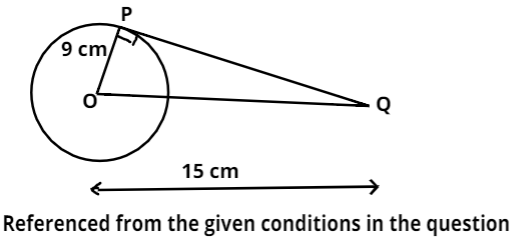Courses
Courses for Kids
Free study material
Free LIVE classes
MoreLIVE
Join Vedantu’s FREE Mastercalss

# Calculate the length of tangent drawn from a point 15 cm away from the centre of the circle of radius 9 cm.Verified
363k+ views
Hint: Let us draw a figure using the given conditions in the question. So, that it will be clearer what should be the tangent to the given circle.So, as we can see from the figure drawn above that OP is the radius of the circle.
And OP = 9 cm.
And Q is the point from which the distance to the centre of the circle is 15 cm (OQ = 15 cm).
So, now PQ will be the tangent drawn from point Q to the circle.
And as we know that from the property of the tangent drawn from any external point to the circles forms a right-angle triangle, with hypotenuse as the line joining the centre of the circle with the external point and the other two sides are radius of circle and the length of tangent .
So, here OPQ will be a right-angled triangle, right-angled at P (point where tangent touches the circle).
Now we had to find the value of PQ.
So, we can apply Pythagoras theorem in the triangle OPQ.
According to Pythagoras theorem, if ABC is any right-angled triangle, right-angled at B. And AC is the hypotenuse of triangle ABC.
Then, ${\left( {{\text{AC}}} \right)^{\text{2}}}{\text{ = }}{\left( {{\text{AB}}} \right)^{\text{2}}}{\text{ + }}{\left( {{\text{BC}}} \right)^{\text{2}}}$
So, applying Pythagoras theorem in triangle OPQ. We get,
${\left( {{\text{OQ}}} \right)^{\text{2}}}{\text{ = }}{\left( {{\text{OP}}} \right)^{\text{2}}}{\text{ + }}{\left( {{\text{PQ}}} \right)^{\text{2}}}$
So, ${\left( {{\text{PQ}}} \right)^{\text{2}}}{\text{ = }}{\left( {{\text{OQ}}} \right)^{\text{2}}}{\text{ - }}{\left( {{\text{OP}}} \right)^{\text{2}}}$
Now as we can see from the above figure that OP = 9 cm and OQ = 15 cm.
So, ${\left( {{\text{PQ}}} \right)^{\text{2}}}{\text{ = }}{\left( {15} \right)^{\text{2}}}{\text{ - }}{\left( 9 \right)^{\text{2}}}{\text{ = 225 - 81 = 144}}$
So, ${\left( {{\text{PQ}}} \right)^{\text{2}}}{\text{ = 144}}$
Now take the square root on both sides of the above equation. We get,
PQ = 12 cm (because length cannot be negative).
Hence, the length of the tangent drawn from a point which is 15 cm away from the centre of the circle is 12 cm.

Note: Whenever we come up with this type of problem where we are asked to find the length of the tangent from an external point then we should use the Pythagoras theorem because tangents drawn from the external point to the circle form a right-angled triangle.
Last updated date: 26th Sep 2023
Total views: 363k
Views today: 3.63k